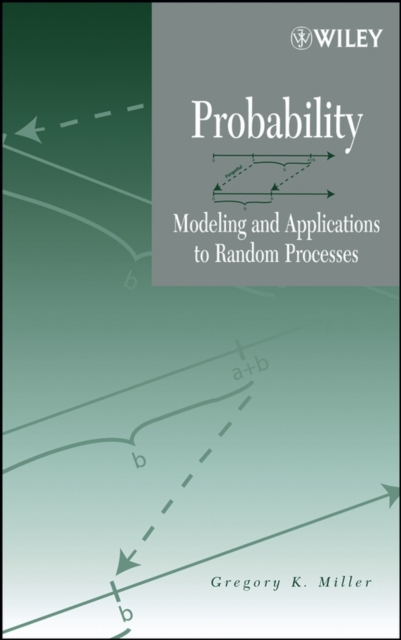# Probability : Modeling and Applications to Random Processes Hardback

Hardback

• Information

#### Description

Improve Your Probability of Mastering This Topic This book takes an innovative approach to calculus-based probability theory, considering it within a framework for creating models of random phenomena.

The author focuses on the synthesis of stochastic models concurrent with the development of distribution theory while also introducing the reader to basic statistical inference.

In this way, the major stochastic processes are blended with coverage of probability laws, random variables, and distribution theory, equipping the reader to be a true problem solver and critical thinker.

Deliberately conversational in tone, Probability is written for students in junior- or senior-level probability courses majoring in mathematics, statistics, computer science, or engineering.

The book offers a lucid and mathematicallysound introduction to how probability is used to model random behavior in the natural world. The text contains the following chapters: Modeling Sets and Functions Probability Laws I: Building on the Axioms Probability Laws II: Results of Conditioning Random Variables and Stochastic Processes Discrete Random Variables and Applications in Stochastic Processes Continuous Random Variables and Applications in Stochastic Processes Covariance and Correlation Among Random Variables Included exercises cover a wealth of additional concepts, such as conditional independence, Simpson's paradox, acceptance sampling, geometric probability, simulation, exponential families of distributions, Jensen's inequality, and many non-standard probability distributions.

£165.00

£125.81